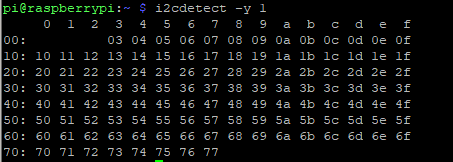saxoalex
Posts: 6
Joined: Mon Dec 02, 2019 6:10 pm

Hi there,
I have some troubles with my raspberry pi 2 B+.
In fact, when I enter the command line 'i2cdetect -y 1' in the console, it shows all addresses, even if nothing is plug to I2C SDA and SCL.I already followed this post : https://www.raspberrypi.org/forums/view ... hp?t=93222. I tried to put a brand new Raspbian without any new softwares. I have the same problem.

I looked for a short circuit between SDA and GND or SCL and GND with a multimeter, but there is no short circuit. All seems correct...

I don't know what to do. I think the problem is hardware.

Can you helm^p me please ?

Thank you.

joan
Posts: 14602
Joined: Thu Jul 05, 2012 5:09 pm
Location: UK

### Re: i2cdetect shows all addresses

SDA is probably shorted to ground.

Run gpiotest.

6by9
Raspberry Pi Engineer & Forum ModeratorPosts: 7770
Joined: Wed Dec 04, 2013 11:27 am
Location: ZZ9 Plural Z Alpha, aka just outside Cambridge.

### Re: i2cdetect shows all addresses

Or you've managed to set the pinmuxing so that two pins are configured to the same SDA function. Check GPIOs 44&45 via "raspi-gpio get".
Software Engineer at Raspberry Pi Trading. Views expressed are still personal views.
I'm not interested in doing contracts for bespoke functionality - please don't ask.

saxoalex
Posts: 6
Joined: Mon Dec 02, 2019 6:10 pm

### Re: i2cdetect shows all addresses

Hi,
I tested gpiotest :And raspi-gpio get :

Code: Select all

``````BANK0 (GPIO 0 to 27):
GPIO 0: level=1 fsel=0 func=INPUT
GPIO 1: level=1 fsel=0 func=INPUT
GPIO 2: level=0 fsel=4 alt=0 func=SDA1
GPIO 3: level=1 fsel=4 alt=0 func=SCL1
GPIO 4: level=0 fsel=0 func=INPUT
GPIO 5: level=0 fsel=0 func=INPUT
GPIO 6: level=0 fsel=0 func=INPUT
GPIO 7: level=0 fsel=0 func=INPUT
GPIO 8: level=0 fsel=0 func=INPUT
GPIO 9: level=0 fsel=0 func=INPUT
GPIO 10: level=0 fsel=0 func=INPUT
GPIO 11: level=0 fsel=0 func=INPUT
GPIO 12: level=0 fsel=0 func=INPUT
GPIO 13: level=0 fsel=0 func=INPUT
GPIO 14: level=1 fsel=4 alt=0 func=TXD0
GPIO 15: level=0 fsel=4 alt=0 func=RXD0
GPIO 16: level=0 fsel=0 func=INPUT
GPIO 17: level=0 fsel=0 func=INPUT
GPIO 18: level=0 fsel=0 func=INPUT
GPIO 19: level=0 fsel=0 func=INPUT
GPIO 20: level=0 fsel=0 func=INPUT
GPIO 21: level=0 fsel=0 func=INPUT
GPIO 22: level=0 fsel=0 func=INPUT
GPIO 23: level=0 fsel=0 func=INPUT
GPIO 24: level=0 fsel=0 func=INPUT
GPIO 25: level=0 fsel=0 func=INPUT
GPIO 26: level=0 fsel=0 func=INPUT
GPIO 27: level=0 fsel=0 func=INPUT
BANK1 (GPIO 28 to 45):
GPIO 28: level=1 fsel=0 func=INPUT
GPIO 29: level=1 fsel=0 func=INPUT
GPIO 30: level=0 fsel=0 func=INPUT
GPIO 31: level=1 fsel=1 func=OUTPUT
GPIO 32: level=0 fsel=1 func=OUTPUT
GPIO 33: level=0 fsel=0 func=INPUT
GPIO 34: level=1 fsel=0 func=INPUT
GPIO 35: level=1 fsel=0 func=INPUT
GPIO 36: level=1 fsel=0 func=INPUT
GPIO 37: level=0 fsel=0 func=INPUT
GPIO 38: level=1 fsel=1 func=OUTPUT
GPIO 39: level=0 fsel=0 func=INPUT
GPIO 40: level=0 fsel=4 alt=0 func=PWM0
GPIO 41: level=0 fsel=1 func=OUTPUT
GPIO 42: level=1 fsel=1 func=OUTPUT
GPIO 43: level=1 fsel=0 func=INPUT
GPIO 44: level=1 fsel=4 alt=0 func=GPCLK1
GPIO 45: level=0 fsel=4 alt=0 func=PWM1
BANK2 (GPIO 46 to 53):
GPIO 46: level=0 fsel=0 func=INPUT
GPIO 47: level=0 fsel=1 func=OUTPUT
GPIO 48: level=0 fsel=4 alt=0 func=SD0_CLK
GPIO 49: level=1 fsel=4 alt=0 func=SD0_CMD
GPIO 50: level=1 fsel=4 alt=0 func=SD0_DAT0
GPIO 51: level=1 fsel=4 alt=0 func=SD0_DAT1
GPIO 52: level=1 fsel=4 alt=0 func=SD0_DAT2
GPIO 53: level=1 fsel=4 alt=0 func=SD0_DAT3
``````

joan
Posts: 14602
Joined: Thu Jul 05, 2012 5:09 pm
Location: UK

### Re: i2cdetect shows all addresses

That indicates GPIO 2, 3, 17, and 18 have failed (assuming nothing was connected to those GPIO during the test).

saxoalex
Posts: 6
Joined: Mon Dec 02, 2019 6:10 pm

### Re: i2cdetect shows all addresses

Nothing was connected.

Have I to connect something to these GPIOs ?

joan
Posts: 14602
Joined: Thu Jul 05, 2012 5:09 pm
Location: UK

### Re: i2cdetect shows all addresses

The GPIO are bust. If something was connected that would have invalidated the test.

saxoalex
Posts: 6
Joined: Mon Dec 02, 2019 6:10 pm

### Re: i2cdetect shows all addresses

Did you meant busy ?

Nothing was connected. What can I conclude ?

trejan
Posts: 1106
Joined: Tue Jul 02, 2019 2:28 pm

### Re: i2cdetect shows all addresses

saxoalex wrote:
Thu Jan 16, 2020 11:58 am
Did you meant busy ?
No. They mean bust the verb for when something is broken.
saxoalex wrote:
Thu Jan 16, 2020 11:58 am
What can I conclude ?
Something has damaged those 4 GPIOs on your Pi. If nothing is connected then the fault is inside the SoC and you need to replace the Pi.

saxoalex
Posts: 6
Joined: Mon Dec 02, 2019 6:10 pm

### Re: i2cdetect shows all addresses

Oh.. Ok..